Oproep: Ik zoek iemand die kan zorgen voor de continuïteit van deze website

Contents[Show/Hide]

# Simulation e-bike hub / mid-drive motor efficiency vs speed

Published: 04 October 2010
Last updated: 04 January 2023
Contents[Show/Hide]

### Intro

Here we describe the simulation of a hub motor on a bicycle in different situations. These different situations are: riding on a flat street, hills and various motor assistance. We create a graph that shows the efficiency versus the speed.

### Bike parameters

Before we can do calculations, the parameters for both the hub motor and the bicycle must be known. Unfortunately the hub motor parameters are seldom known. The bicycle parameters have to estimated or calculated. These are the e-bike parameters, some values were estimated:

 Total mass 90 [kg] Rolling resistance Cr 0.005 Air density ρ 1.23 Drag coefficient Cw 1 Reference area 0,5 [m²] Transmission efficiency (hub motor) 100 [%] Wheel diameter 0.688 [m]

### Motor parameters

Commonly, instead of motor parameters, a graph like this is included with a hub motor: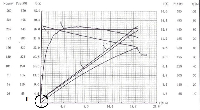Common electric motor torque / speed efficiency curve

This graph is of no help because the values are plotted in relationship with the torque whereby the voltage is constant. But on a bike, because of the air drag, the load is speed dependant. The motor voltage increases with the speed.

See here how we can extract motor parameters from the graph.  These are the hub motor parameters extracted from the above graph:

• R* = 0.6Ω (includes the gearbox loss)
• k = 1.57
• Friction torque Tf = 0.82Nm

Now, when we know all the parameters, the motor-bicycle combination can be simulated in Excel.

### Simulation

Here is an Excel graph of an e-bike with a hub motor, riding on a flat street from 0 to 30 kmh and 100% motor assistance.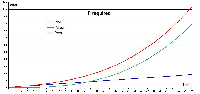Required power, slope = 0 degree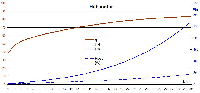E-bike hub motor graph efficiency vs speed, slope = 0 degreeCurrent, slope = 0 degree

We see that the higher the power, the higher the efficiency. At low speeds the efficiency is low. But remember that this is not dramatically because the power here is low and the loss thus of minor importance.

Note that the motor parameters are acquired on a different way here, which delivers slightly different graphs.

In this Excel sheet on GitHub you can fill in your motor and bike parameters, the slope and the amount of motor assistance. Or, if you want, use EPACSim.

### EPACSim

EPACSim is a free program to simulate a motor on a bicycle in different situations.

Here are some applications

• Simulation of different motors / controller / battery.
• Comparison of similar motors. Identification of damage / problems on the basis of comparative measurements.
• Deepening of practical knowledge relevant to the operation of BLDC motors.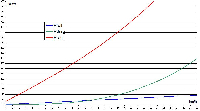Required power, slope = 5 degree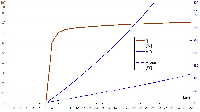E-bike hub motor curve efficiency vs speed, slope = 5 degree